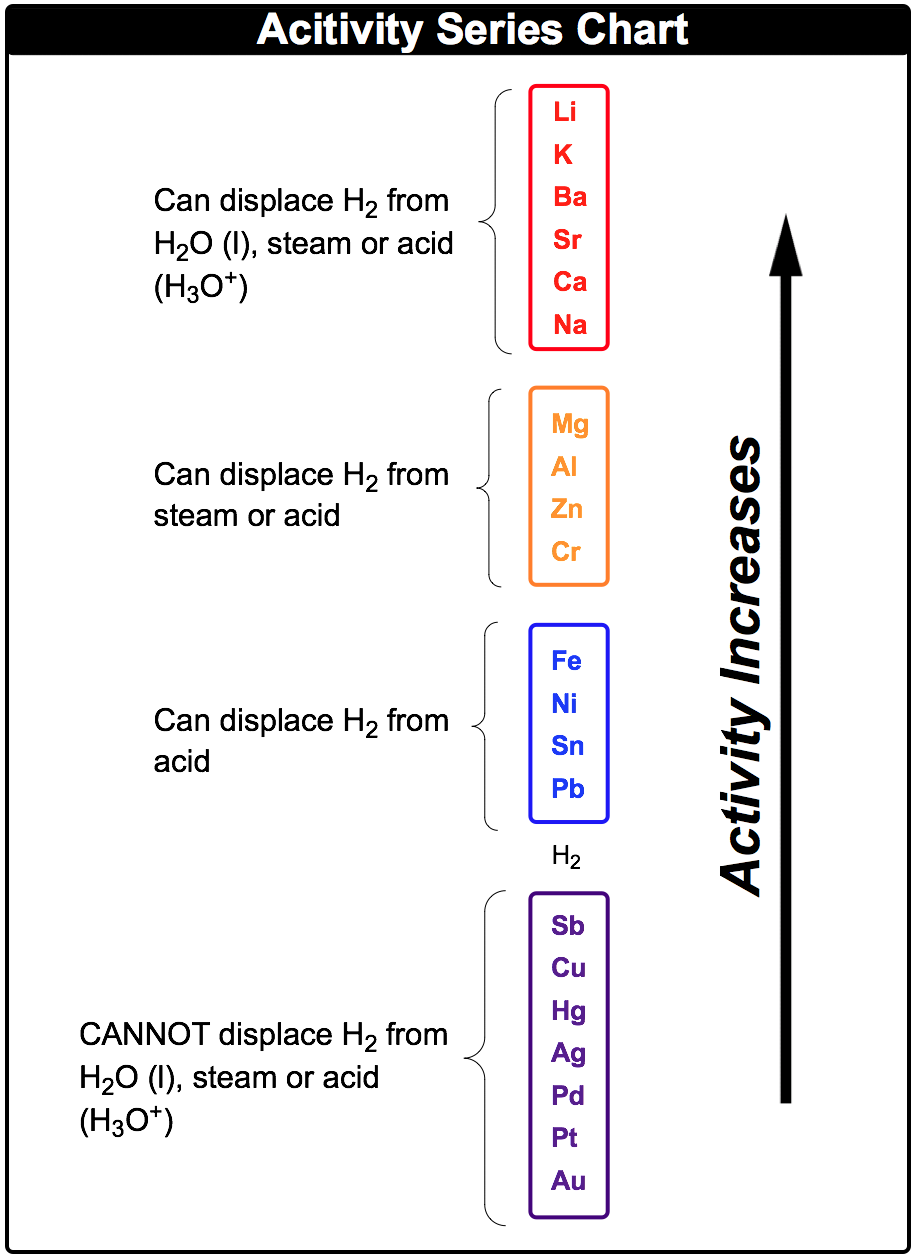Clutch Prep is now a part of Pearson
Ch.4 - Chemical Quantities & Aqueous ReactionsWorksheetSee all chapters

# Activity Series

See all sections
Sections
Solutions
Molarity
Osmolarity
Dilutions
Solubility Rules
Electrolytes
Molecular Equations
Gas Evolution Equations
Solution Stoichiometry
Complete Ionic Equations
Calculate Oxidation Numbers
Redox Reactions
Balancing Redox Reactions: Acidic Solutions
Balancing Redox Reactions: Basic Solutions
Activity Series

Activity Series is a list of single displacement reactions in order of reactivity.

###### The Activity Series Chart

Concept #1: Activity Series

Concept #2: Activity Series ChartExample #1: A very common example is the displacement of a hydrogen atom by magnesium when reacting with hydrochloric acid. Based on your understanding of the activity series chart, determine the products formed.

Practice: Based on your understanding of activities determine if a reaction occurs and if so provide the products formed.

Ba (s) + H2O (g) →

Practice: Based on your understanding of activities determine if a reaction occurs and if so provide the products formed.

Zn (s) + NiCl2 (aq) →

Practice: If the activity of halogens is stated as: Fluorine > Chlorine > Bromine > Iodine, determine if a reaction occurs and if so provide the products formed.

Cl2 (g) + AlBr3 (aq) →